# 基于细分曲面边界元法的声学分析Acoustic Analysis Based on Isogeometric Boundary Element Method with Subdivision Surfaces

DOI: 10.12677/OJAV.2019.74020, PDF, HTML, XML, 下载: 408  浏览: 1,302

Abstract: The core idea of subdivision surfaces is to repeatedly refine the initial polygon mesh and obtain smooth limit surfaces. The Catmull-Clark subdivision surface is developed based on the bicubic B-spline and is suitable for subdivision and fitting operations on arbitrary quadrilateral meshes. Combining the subdivision surface with the boundary element method, spline interpolation is applied to both geometry and physics in numerical analysis, which ensures the geometric accuracy of the analysis model and improves the accuracy of physical interpolation. In this paper, a Cat-mull-Clark based on subdivision surface method and a Catmull-Clark based on acoustic boundary element method are used to establish an extremely smooth spherical model, and the model is used to analyze the acoustic scattering response when plane waves are incident to prove the accuracy of the CCBEM method.

1. 引言

2. 基于Catmull-Clark的细分曲面方法

2.1. Catmull-Clark细分规则

CC (Catmull-Clark)是将任意四边形网格细分为四个子四边形网格的算法 。k级网格细分一次生成三种顶点：1、原始网格根据周围顶点权重获得顶点V；2、在任意四边形单元的各边的中部插入E顶点；3、在任意四边形单元的中心位置插入F顶点。三顶点的计算表达式如下  ：(如图1所示)

1) ${V}^{k\text{+}1}$ 顶点坐标为：

${X}_{iv}^{k+1}=\gamma {X}_{iv}^{k}+\frac{\alpha }{v}\underset{i=1}{\overset{v}{\sum }}{X}_{2i-1,iv}^{k}+\frac{\beta }{v}\underset{i=1}{\overset{v}{\sum }}{X}_{2i,iv}^{k}$ (1)

$\alpha =\frac{3}{2v}$$\beta =\frac{1}{4v}$$\gamma =1-\alpha -\beta$${X}_{2i-1,iv}^{k}$${X}_{2i,iv}^{k}$ 为相邻顶点坐标。

2) ${E}^{k+1}$ 顶点坐标为：

${X}_{ie}^{k+1}=\frac{3}{8}\left({X}_{1,ie}^{k}+{X}_{2,ie}^{k}\right)+\frac{1}{16}\left({X}_{3,ie}^{k}+{X}_{4,ie}^{k}+{X}_{5,ie}^{k}+{X}_{6,ie}^{k}\right)$ (2)

${X}_{1,ie}^{k}$${X}_{2,ie}^{k}$${X}_{3,ie}^{k}$${X}_{4,ie}^{k}$${X}_{5,ie}^{k}$${X}_{6,ie}^{k}$ 为相邻顶点的坐标。

3) ${F}^{k+1}$ 顶点坐标为：

${X}_{if}^{k+1}=\frac{1}{4}\left({X}_{1,if}^{k}+{X}_{2,if}^{k}+{X}_{3,if}^{k}+{X}_{4,if}^{k}\right)$ (3)

${X}_{1,if}^{k}$${X}_{2,if}^{k}$${X}_{3,if}^{k}$${X}_{4,if}^{k}$ 为四边形单元的坐标。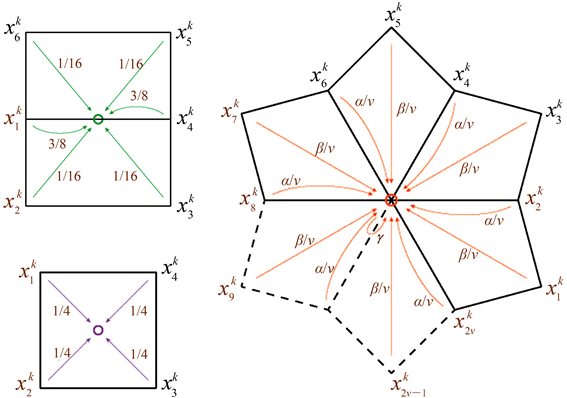Figure 1. Distribution of edge point “E”, midpoint “F” and vertex point “V”

2.2. 曲面拟合

2.2.1. 规则单元曲面拟合

${X}^{e}\left({\theta }_{1},{\theta }_{2}\right)=\underset{i=1}{\overset{16}{\sum }}B\left({\theta }_{1},{\theta }_{2}\right){X}_{i}$ (4)

${X}_{i}$ 为第i个相邻顶点的坐标，参数域满足 $\left\{\left({\theta }_{1},{\theta }_{2}\right)|{\theta }_{1}\in \left(0,1\right),{\theta }_{2}\in \left(0,1\right)\right\}$$B\left({\theta }_{1},{\theta }_{2}\right)$ 为双三次B样条基函数

$6{N}_{0}\left(t\right)=1-3t+3{t}^{2}-{t}^{3}$ (5)

$%$ 为余数， $/$ 为除法，N为三次B样条基函数

$6{N}_{0}\left(t\right)=1-3t+3{t}^{2}-{t}^{3}$ (6)

$6{N}_{1}\left(t\right)=4-6{t}^{2}+3{t}^{3}$ (7)

$6{N}_{2}\left(t\right)=1+3t+3{t}^{2}-3{t}^{3}$ (8)

$6{N}_{3}\left(t\right)={t}^{3}$ (9)

2.2.2. 不规则单元的曲面拟合

${X}_{n,k}\left({\theta }_{1},{\theta }_{2}\right)=\underset{i=0}{\overset{16}{\sum }}B\left({\stackrel{¯}{\theta }}_{1},{\stackrel{¯}{\theta }}_{2}\right){X}_{n,k}=B\left({\stackrel{¯}{\theta }}_{1},{\stackrel{¯}{\theta }}_{2}\right){X}_{n,k}=B\left({\stackrel{¯}{\theta }}_{1},{\stackrel{¯}{\theta }}_{2}\right){P}_{n}{\stackrel{¯}{C}}_{k}$ (10)

${P}_{n}$ 为拾取矩阵， $\left({\theta }_{1},{\theta }_{2}\right)$ 为初始拟合点x的坐标， $\left({\stackrel{¯}{\theta }}_{1},{\stackrel{¯}{\theta }}_{2}\right)$ 为细分后规则子单元中拟合点x的坐标。

${X}^{e}\left({\theta }_{1},{\theta }_{2}\right)\text{=}\underset{i=1}{\overset{2v+8}{\sum }}{B}^{ir}\left({\theta }_{1},{\theta }_{2}\right){X}_{i}^{ir}={B}^{ir}{C}_{0}$ (11)

${X}_{i}^{ir}$ 是第i个相邻顶点的坐标， ${B}^{ir}\left({\theta }_{1},{\theta }_{2}\right)$ 是不规则单元的基函数， ${B}^{ir}$ 是基函数矩阵

${B}^{ir}=B\left(t,k\left({\theta }_{1},{\theta }_{2}\right)\right){P}_{n}\stackrel{¯}{A}{A}^{k-1},n=1,2,3$ (12)

3. 基于Catmull-Clark的声学边界元法

3.1. 声学边界积分公式

${\nabla }^{2}p\left(x\right)+{k}^{2}p\left(x\right)=0,\text{\hspace{0.17em}}\text{\hspace{0.17em}}\forall x\in \Omega$ (13)

$\nabla$ 为拉普拉斯算子， $p\left(x\right)$ 为点x处的声压，波数为 $k=\omega /c$$\omega$ 为角频率，c为传播流体域中的声波速度。对公式(13)进行一系列转换后，可以得到以下边界积分方程

$C\left(x\right)p\left(x\right)+\left({H}^{*}p\right)\left(x\right)=\left({G}^{*}q\right)\left(x\right)+{p}_{inc}\left(x\right)$ (14)

${p}_{inc}$ 为入射波声压， ${G}^{*}$ 和v为边界积分算子。

$C\left(x\right)q\left(x\right)+{\int }_{\Gamma }\frac{{\partial }^{2}G\left(x,y\right)}{\partial n\left(x\right)\partial n\left(y\right)}p\left(y\right)\text{d}\Gamma \left(y\right)={\int }_{\Gamma }\frac{\partial G\left(x,y\right)}{\partial n\left(x\right)}q\left(y\right)\text{d}\Gamma \left(y\right)+\frac{\partial {p}_{inc}\left(x\right)}{\partial n\left(x\right)}$ (15)

$\begin{array}{l}C\left(x\right)\left[p\left(x\right)+\alpha q\left(x\right)\right]+\left({H}^{*}p\right)\left(x\right)+\alpha \left({\stackrel{¯}{H}}^{*}p\right)\left(x\right)\\ =\left({G}^{*}q\right)\left(x\right)+\alpha \left({\stackrel{¯}{G}}^{*}q\right)\left(x\right)+{p}_{inc}\left(x\right)+\alpha {\stackrel{¯}{p}}_{inc}\left(x\right)\end{array}$ (16)

$\alpha$ 为组合参数：当 $k>1$ 时， $\alpha =i/k$，否则 $\alpha =i$

3.2. 离散化

$\begin{array}{l}{p}_{e}=\underset{n=1}{\overset{2v+8}{\sum }}{B}_{n}\left({\theta }_{1},{\theta }_{2}\right){p}_{n}^{e}\\ {q}_{e}=\underset{n=1}{\overset{2v+8}{\sum }}{B}_{n}\left({\theta }_{1},{\theta }_{2}\right){q}_{n}^{e}\end{array}$ (17)

${p}_{e}$${q}_{e}$${\Gamma }_{e}$ 单元内点 $\left({\theta }_{1},{\theta }_{2}\right)$ 的声压、法线通量， ${p}_{n}^{e}$${q}_{n}^{e}$${\Gamma }_{e}$ 单元网格中某节点的声压、法线通量。

$\begin{array}{l}C\left(x\right)\left(p\left(x\right)+\alpha q\left(x\right)\right)\\ ={p}_{i}\left(x\right)+\alpha \frac{\partial {p}_{i}\left(x\right)}{\partial n\left(x\right)}+\underset{e=1}{\overset{{N}_{e}}{\sum }}\underset{n=1}{\overset{2v+8}{\sum }}{q}_{n}^{e}{\int }_{{\Gamma }_{e}}{B}_{n}\left({\theta }_{1},{\theta }_{2}\right)G\left(x,y\left({\theta }_{1},{\theta }_{2}\right)\right)\text{d}\Gamma \\ \text{\hspace{0.17em}}\text{\hspace{0.17em}}-\underset{e=1}{\overset{{N}_{e}}{\sum }}\underset{n=1}{\overset{2v+8}{\sum }}{p}_{n}^{e}{\int }_{{\Gamma }_{e}}{B}_{n}\left({\theta }_{1},{\theta }_{2}\right)\frac{\partial G\left(x,y\left({\theta }_{1},{\theta }_{2}\right)\right)}{\partial n\left(y\left({\theta }_{1},{\theta }_{2}\right)\right)}\text{d}\Gamma \\ \text{\hspace{0.17em}}\text{\hspace{0.17em}}+\alpha \underset{e=1}{\overset{{N}_{e}}{\sum }}\underset{n=1}{\overset{2v+8}{\sum }}{q}_{n}^{e}{\int }_{{\Gamma }_{e}}{B}_{n}\left({\theta }_{1},{\theta }_{2}\right)\frac{\partial G\left(x,y\left({\theta }_{1},{\theta }_{2}\right)\right)}{\partial n\left(x\right)}\text{d}\Gamma \\ \text{\hspace{0.17em}}\text{\hspace{0.17em}}-\alpha \underset{e=1}{\overset{{N}_{e}}{\sum }}\underset{n=1}{\overset{2v+8}{\sum }}{p}_{n}^{e}{\int }_{{\Gamma }_{e}}{B}_{n}\left({\theta }_{1},{\theta }_{2}\right)\frac{{\partial }^{2}G\left(x,y\left({\theta }_{1},{\theta }_{2}\right)\right)}{\partial n\left(x\right)\partial n\left(y\left({\theta }_{1},{\theta }_{2}\right)\right)}\text{d}\Gamma \end{array}$ (18)

$Hp=Gq+{p}_{inc}$ (19)

H、G是CCBEM的系数矩阵，p、 ${p}_{inc}$ 是配置点处的声压和入射波矩阵。

4. 数值例子(a) 初始网格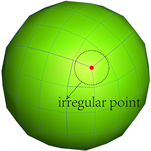(b) 1级细分网格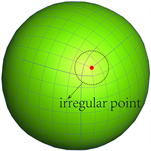(c) 2级细分网格(d) 3级细分网格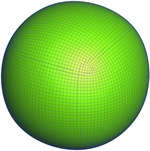(e) 4级细分网格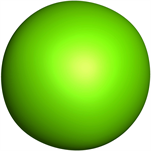(f) 极限细分网格

Figure 2. Initial mesh and multilevel subdivision meshes: (a) initial mesh with 24 elements and 26 nodes; (b) 1 level of subdivision surface with 98 nodes and 96 elements; (c) 2 level of subdivision surface with 386 nodes and 384 elements; (d) 3 level of subdivision surface with 1538 nodes and 1536 elements; (e) 4 level of subdivision surface with 6146 nodes and 6144 elements; (f) limited subdivision surface with 98,306 nodes and 98,304 element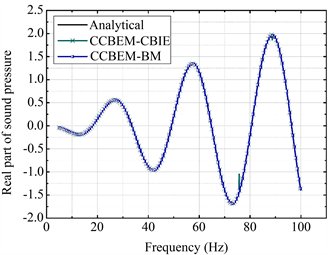(a) 实部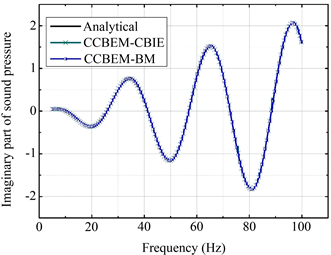(b) 虚部

Figure 3. Comparisons between numerical and analytical solutions of sound pressure at a point of (10;0;0): (a) Real part of sound pressure as a function of frequency; (b) Imaginary part of sound pressure as a function of frequency. The frequency step is 0.1 Hz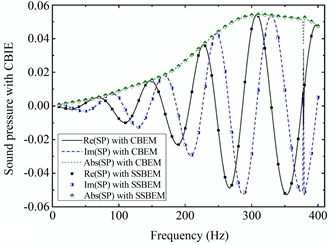(a) CBIE的声压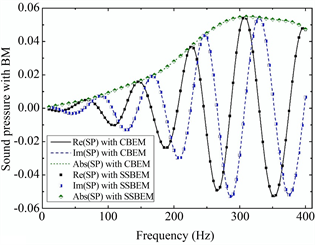(b) BM的声压

Figure 4. Changes in the real, imaginary, and amplitude of the sound pressure at point (2; 0; 0) with frequency: (a) CBIE is used for numerial solution; (b) BM is used for numerial solution. Black solid line denotes the real part of sound pressure with CBEM; Dash line denotes the imaginary part of sound pressure with CBEM; Dot line denotes the amplitute of sound pressure with CBEM; Three different types of scatters are used to represent the solution by CCBEM

$\frac{\partial p}{\partial n}={\frac{\partial p}{\partial r}|}_{r=a}=-Ak{j}_{1}\left(ka\right)=i\omega \rho {v}_{0}$ (20)

$A=-\frac{i\omega \rho {v}_{0}}{k{j}_{1}\left(ka\right)}$ (21)

$p\left(r\right)=-\frac{i\omega \rho {v}_{0}}{k}\frac{{j}_{0}\left(kr\right)}{{j}_{1}\left(ka\right)}$ (22)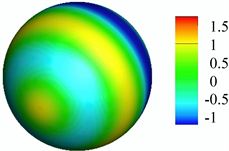(a) 50 HZ时的实部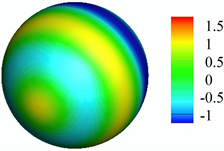(b) 50 HZ时的虚部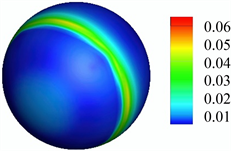(c) 50 HZ时的曲面误差(d) 100 HZ时的实部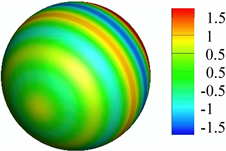(e) 100 HZ时的虚部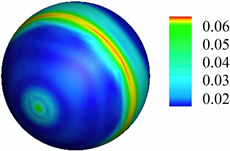(f) 100 HZ时的曲面误差

Figure 5. Distribution of sound pressure and surface error on limited spherical surface:(a) Real part of sound pressure at 50 Hz; (b) Imaginary part of sound pressure at 50 Hz; (c) Surface error at 50 Hz; (d) Real part of sound pressure at 100 Hz; (e) Imaginary part of sound pressure at 100 Hz;(f) Surface error at 100 Hz

5. 总结

  Pan, Q., Xu, G.L. and Zhang, Y.J. (2013) A Unified Method for Hybrid Subdivision Surface Design Using Geometric Partial Differential Equations. Computer-Aided Design, 46, 110-119. https://doi.org/10.1016/j.cad.2013.08.023  Hughes, T., Reali, A. and Sangalli, G. (2010) Efficient Quadrature for NURBS-Based Isogeometric Analysis. Computer Methods in Applied Mechanics and Engineering, 199, 301-313. https://doi.org/10.1016/j.cma.2008.12.004  Jüttler, B., Mantzaflaris, A., Perl, R. and Rumpf, M. (2016) On Numer-ical Integration in Isogeometric Subdivision Methods for PDEs on Surfaces. Computer Methods in Applied Mechanics and Engineering, 302, 131-146. https://doi.org/10.1016/j.cma.2016.01.005  Shen, J.J., Kosinka, J., Sabin, M. and Dodgson, N. (2014) Conversion of Trimmed NURBS Surfaces to Catmull-Clark Subdivision Surfaces. Computer Aided Geometric Design, 31, 486-498. https://doi.org/10.1016/j.cagd.2014.06.004  Wawrzinek, A. and Polthier, K. (2016) Integration of Generalized B-Spline Functions on Catmull-Clark Surfaces at Singularities. Computer-Aided Design, 78, 60-70. https://doi.org/10.1016/j.cad.2016.05.008  Wei, X.D., Zhang, Y.J., Hughes, T.J.R. and Scott, M.A. (2015) Trun-cated Hierarchical Catmull-Clark Subdivision with Local Refinement. Computer Methods in Applied Mechanics and En-gineering, 291, 1-20. https://doi.org/10.1016/j.cma.2015.03.019  Pan, Q., Xu, G.L., Xu, G. and Zhang, Y.J. (2016) Isogeometric Analy-sis Based on Extended Catmull-Clark Subdivision. Computers and Mathematics with Applications, 71, 105-119. https://doi.org/10.1016/j.camwa.2015.11.012  Oden, J.T., Prudhomme, S. and Demkowicz, L. (2005) A Posteriori Error Estimation for Acoustic Wave Propagation Problems. Archives of Computational Methods in Engineering, 12, 343-389. https://doi.org/10.1007/BF02736190  Chen, L., Zheng, C. and Chen, H. (2014) FEM/Wideband FMBEM Coupling for Structural-Acoustic Design Sensitivity Analysis. Computer Methods in Applied Mechanics and Engineer-ing, 276, 1-19. https://doi.org/10.1016/j.cma.2014.03.016  郑昌军. 三维结构声学及其敏感度分析的宽度快速多级边界元算法研究[D]: [博士学位论文]. 合肥: 中国科学技术大学, 2011.